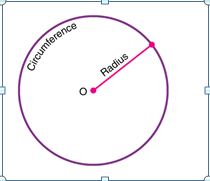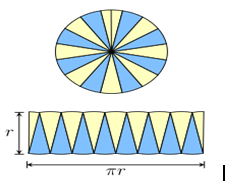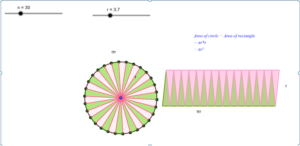# Area of the circle using the derivatives of the rectangle in GeoGebra.

Area of circle:

The area of a circle is the region covered or enclosed within its boundary. It is measured in square units. The area covered by one complete cycle of the radius of the circle on a two-dimensional plane is the area of that circle.

Area of circle formula:Let us take a circle with a radius r from the center ‘o’ to the boundary of the circle. Then the area for this circle, A, is equal to the product of pi and the square of the radius. It is given by;

Area of a Circle, A = πr2 square units

Here, the value of pi, π = 22/7 or 3.14, and r is the radius.

Deriving the area of the circle:

The area of a circle can be visualized & proved using two methods, namely

• Determining the circle’s area using rectangles.
• Determining the circle’s area using triangles.

Using the area of a rectangle:

The circle is divided into equal sectors, and the sectors are arranged as shown in fig. 3. The area of the circle will be equal to that of the parallelogram-shaped figure formed by the sectors cut out from the circle. Since the sectors have equal areas, each sector will have an equal arc length. The blue coloured sectors will contribute to half of the circumference, and the yellow-coloured sectors will contribute to the other half. If the number of sectors cut from the circle is increased, the parallelogram will eventually look like a rectangle with length equal to πr and breadth equal to r.

The area of a rectangle (A) will also be the area of a circle. So, we have

• A = π×r×r
• A = πr2Let’s see the practical execution of the area of the circle using the derivatives of the rectangle in GeoGebra.

Step 1: By using a slider operation, create a number slider (n) with the following values.

• Set Min – 1; Max- 100 and increment as 1.

Step 2: By using a slider operation, create a radius slider (r) with the following values.

• Set Min – 4; Max- 10 and increment as 0.1.

Step 3: Plot a point A and draw a circle with radius r by keeping A as the center.

Step 4: Plot a point B anywhere on the circle.

Step 5: Give input as Rotate (B,360°/n, A).

Step 6: Now point B’ appears.

Step 7: Draw a segment between B and B’.

Step 8: Plot the midpoint C and draw a segment connecting the center and point C.

Step 9: Give the following set of inputs:

• List1=Sequence (Rotate(B,j(360°)/n,A),j,0,n).
• List2=Sequence (circularsector (A, Element (List1, j), Element (List1,j+1)),j,1,n,2).
• List3=Sequence (circularsector (A, Element (List1, j), Element (List1,j+1)),j,2,n,2).
• List4=Sequence(circularsector((jf,0), (jf+f/2,g)(jf+(-f)/2,g))),j,0,n/2-1).
• List5=Sequence (circularsector((jf+f/2,g),(jf+0,0),(jf+f,0))),j,0,n/2-1).

Step 10: Now we can observe the sectors getting formed and listed as per the input.

Step 11: Colour the sectors accordingly and insert the text for the area of the rectangle.

Step 12: By moving the number slider we observe the desired output. i.e., Area thus formed by the sectors forms a rectangle.https://www.geogebra.org/calculator/sczjra48